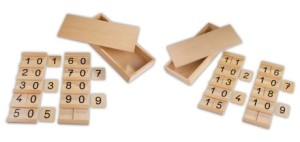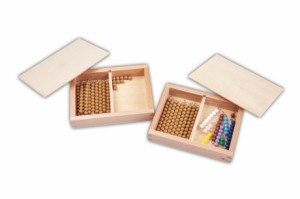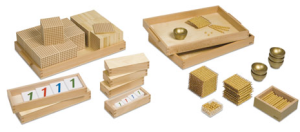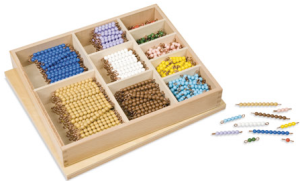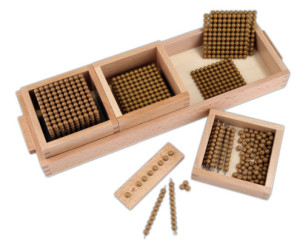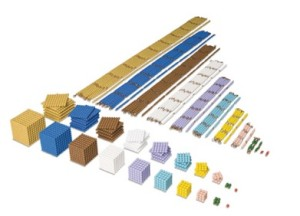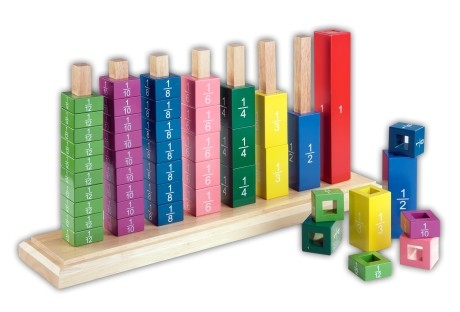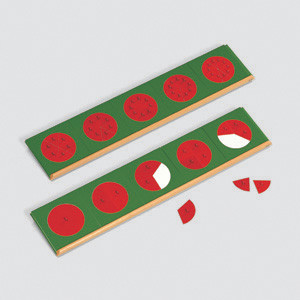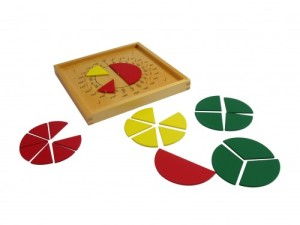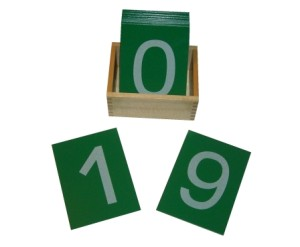Maths

Mathematics is one of the most useful and fascinating divisions of human knowledge. It is used in many important areas of study, e.g. science, architecture, statistics etc. It is the study of quantities and relations through the use of numbers and symbols. We use mathematics daily, even in such simple ways as telling the time or counting change.

The sensorial materials are an indirect preparation for the maths programme; they aid the later transition to abstract thinking by providing a firm foundation upon which to build abstract thought. Our society uses a decimal system for counting (counting in tens). The child is given a sensorial impression of this system from his/her early days in school: all the early sensorial materials for dimension are in sets of ten.

There is a vast difference between the mere acquisition of mathematical facts and the creative growth of mathematical understanding. A child learns best by doing, and therefore, the learning experience should be as concrete as possible. S/he should be afforded opportunities to explore mathematics by the use of concrete materials, so that s/he develops the concepts of mathematics in a meaningful way through his/ her own activity.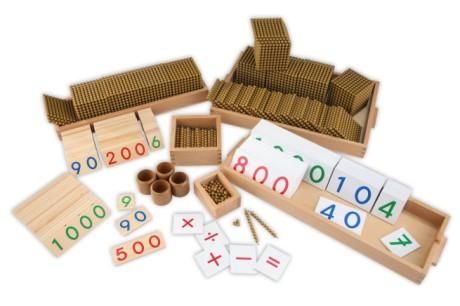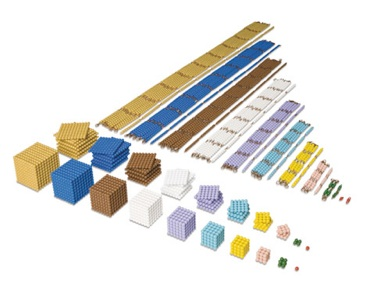### Skills developed / Purposes of Maths Exercises

The Maths programme benefits the child in many ways, some of which are
outlined below: –

• Effective use of mathematical language
• Recognition of mathematical symbols
• Use of mathematical equipment/materials
• Introduction to the decimal system
• Introduction to mathematical operations
• Introduction to sequence, pattern etc.

### Syllabus/ Programme Content

• Classifying
• Matching
• Comparing
• Ordering

#### Shape and Space

• Spatial awareness
• Position (over, under, on etc.)
• Directions (left, right, straight etc.)

• Length
• Weight
• Capacity
• Time
• Money

#### Data

• Recognising and interpreting data
• Sorting collections according to a specific (colour, shape, size)
• Pictograms (bar graphs etc.)

#### Number

##### Numbers, 0 – 10
• The number rods
• The sandpaper numerals
• Number rods and cards
• The spindle box
• Cards and counters
• Number games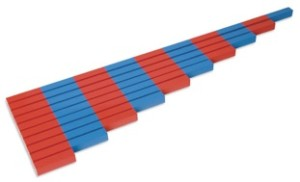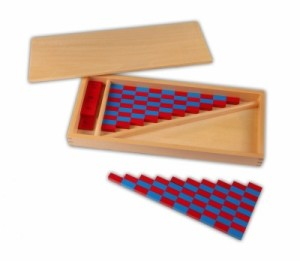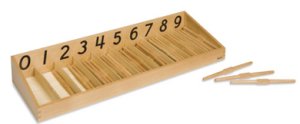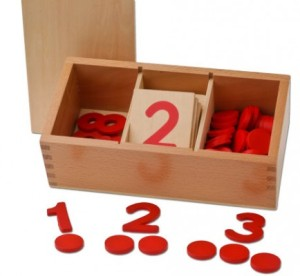#### Quantity

• Naming the hierarchy (1, 10, 100, 1000)
• Counting through
• Recognising quantities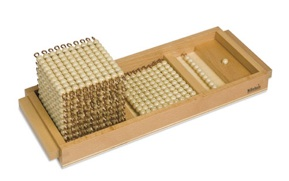#### Symbol

• Naming the symbols (1, 10, 100, 1000)
• Counting through
• Recognising symbols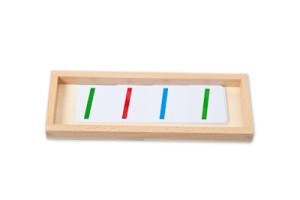#### Quantity & Symbol

• The Bird’s Eye View
• Combining quantity and symbol
• The Changing Game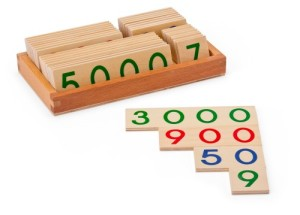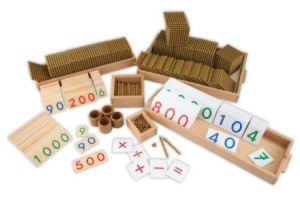#### 11 – 19

• Naming the quantities, 11-19
• Naming the written symbols, 11-19
• Combining quantities and written symbols, 11-19